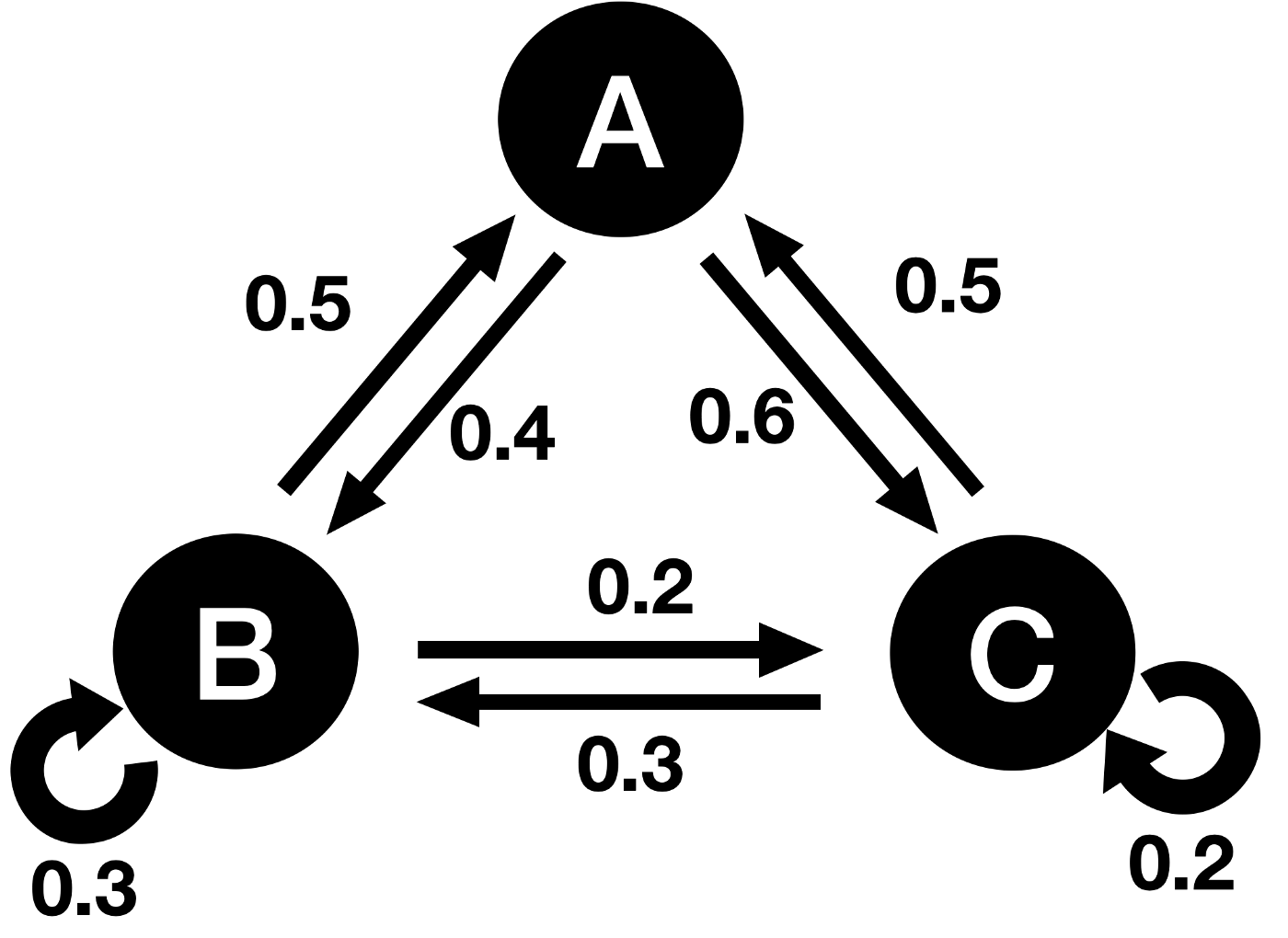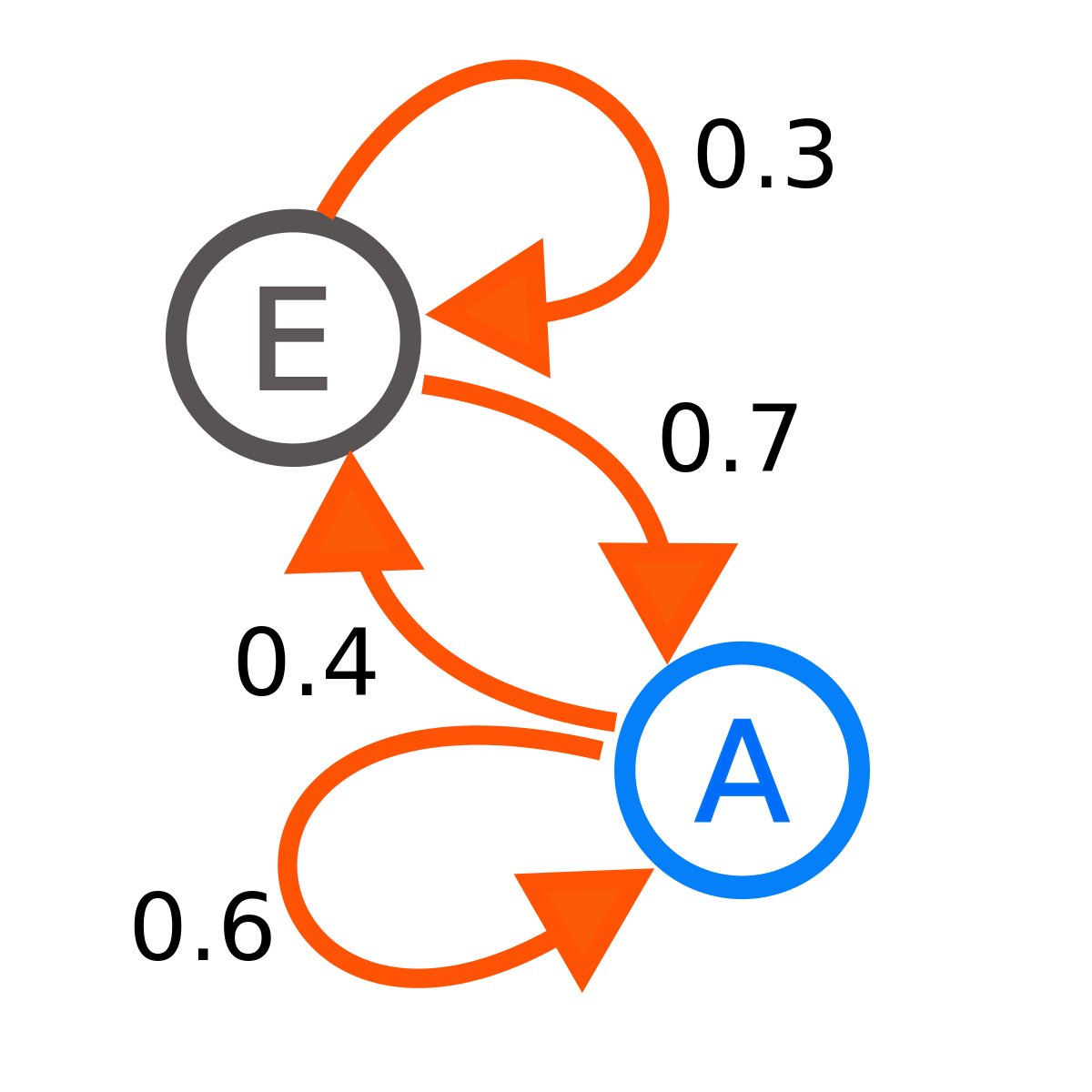##### Markov chain

The stochastic model describes a series of possible events in which each event's probability depends only on the previous event's state.

A …

Oct. 19, 2022 1 minuteThe stochastic model describes a series of possible events in which each event's probability depends only on the previous event's state.

A Markov chain, named after the Russian mathematician Andrey Markov, is a mathematical system in which your states change from one to another.

In a Markov chain, the conditional probability distribution for the next state depends only on the current state and is independent of the past form. The Markov property describes this memorylessness.

There are many applications of Markov chains in real-world modeling.## What is a Markov chain?

Markov processes satisfy the Markov property (sometimes called memorylessness). It is a process for which predictions regarding future outcomes are possible based solely on its present state.

Most importantly, these predictions are as accurate as those based on their entire history. Therefore, the system's future and past states are independent of its present form.

Markov chains may have a discrete state space or a discrete index set (often representing time), but the precise definition varies.

A Markov chain is typically defined as a Markov process in discrete or continuous time with a countable state space (thus, regardless of the nature of time).

Although this is true, Markov chains are commonly defined as having discrete time in either a countable or continuous state space (thus regardless of the state space).

## How to find stationary distribution Markov chain?

In a Markov chain, a stationary distribution is a probability distribution that remains unchanged over time. In general, it is represented as a row vector π whose entries are probabilities summing to 1 and given a transition matrix P; it satisfies

π = πP

In other words, π is invariant by the matrix P.

## What is the Markov chain used for?

Speech recognition: In most automatic speech recognition systems, hidden Markov models are used.

Statistics: Markov chain methods have become very important for generating sequences of random numbers to accurately reflect complicated desired probability distributions Markov chain Monte Carlo (MCMC).

Social sciences: Markov chains are commonly used to describe path-dependent arguments in which current structural configurations influence future outcomes.

Games: A Markov chain can be used to model various chance games. Snakes and ladders and "Hi Ho! Cherry-O" are both represented precisely by Markov chains.

Music: Markov chains are used in algorithmic music composition, especially in programs such as Csound, Max, and SuperCollider.

General Hygiene: A Markov chain mathematically models future disease outbreaks in public health. These models identify each person with their current state (having or not having a disease).## Conclusion

Markov and hidden Markov models are used in various fields, especially artificial intelligence. These models can recognize handwriting, speech, and signatures and determine musical patterns.

Econometrics, queueing theory, and the detection of financial patterns are also fields that involve Markov models. Markovian stochastic processes and hidden Markovian models are, therefore, widely used.